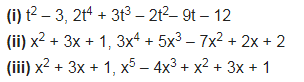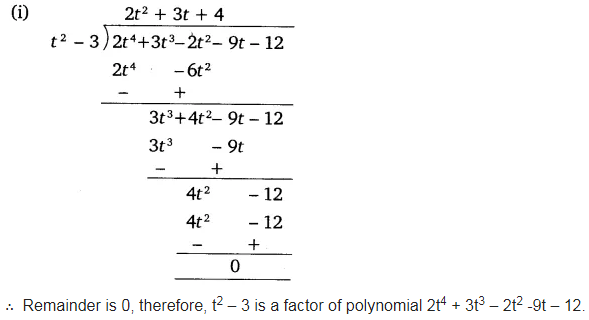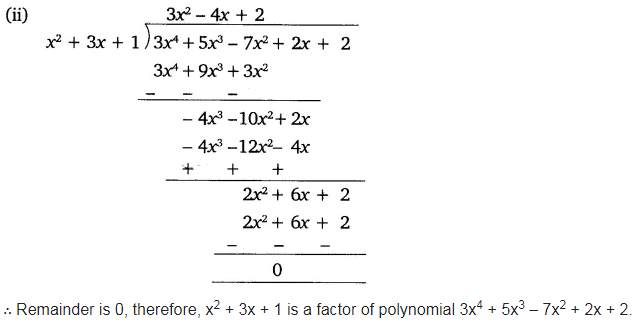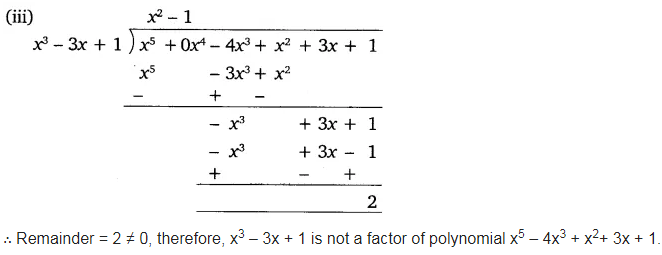# Check whether the first polynomial is a factor of the second polynomial by dividing the second polynomial

Check whether the first polynomial is a factor of the second polynomial by dividing the second polynomial by the first polynomial.